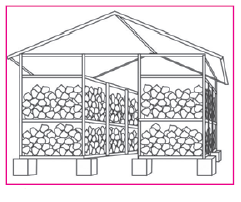Share

# Quadratic Equations Examples and Solutions

#### notes

Quadratic equations are useful in daily life for finding solutions of some practical problems. We are now going to learn the same.
Ex. (1) There is a rectangular onion storehouse in the farm of Mr. Ratnakarrao at Tivasa. The length of rectangular base is more than its breadth by 7 m and diagonal is more than length by 1 m. Find length and breadth of the storehouse.

Solution : Let breadth of the storehouse be x m.
∴length = (x + 7) m, diagonal = x + 7 + 1 = (x + 8) m
By Pythagorus theorem

x^2+(x+7)^2=(x+8)^2

x^2+x^2+14x+49=x^2+16x+64

therefore x^2+14x-16x+49-64=0

therefore x^2-2x-15=0

therefore x^2-5x+3x-15=0

therefore x(x-5)+3(x-5)=0

therefore (x-5) (x+3)=0

therefore x-5=0 or x+3=0

therefore x=5 or x=-3

But length is never negative ∴x≠-3

∴x = 5 and x + 7 = 5 + 7 = 12

Length of the base of storehouse is 12m and breadth is 5m.### Shaalaa.com

Quadratic Equations Part 08 Word Problems [00:23:10]
S
0%